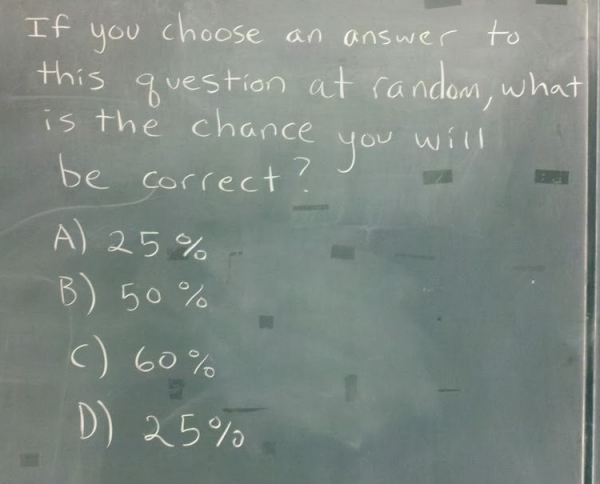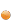## Friday, October 28, 2011Problem?

viaIf you liked this post, you can subscribe to the Blame It On The Voices RSS feed and get your regular fix

#### 11 comentarii:

Anonymous said...

B.

Anonymous said...

@Anon, No, because if you'd be right 50% of the time, then half of the answers must be 50%.

Anonymous said...

True

Pablo Nuñez said...

25%

Anonymous said...

B.

Anonymous said...

if two out of four answers are the same, then neither is correct.
so only B and C are possible.
so that's 50/50.
therefore, B.

Anonymous said...

Because 25% is listed twice, you have a 50% chance to be correct making B the right answer, therefore reducing the chance to be correct to 25% making A & D the right answers, therefore raising the chance to be correct to 50% and so and so... It is a paradox and the chance that you will be correct is 0% regardless the 4 choices and the percentage they show. After all, the question asks for a percentage as a final output, it doesn't limit you to the 4 choices as the only answers.

Anonymous said...

this is similar to the liar-paradox

Anonymous said...

What question? They don't ask you a question, so how can you determine the probability of being right? None of the answers is possible.

rishabh sharma said...

then
let me start from the beginning
we don't know which of these is the correct answer, so the probability of the answer being A (or D as they have same values) is 1/3 and the probability of us choosing A is 1/4 thus the probability of us being correct if answer is A is 1/3*1/4. similar applies for B,C and D. summing the probabilities we get (1/3*1/4)+(1/3*1/4)+(1/3*1/4)+(1/3*1/4= 1/3 or 33.33%.
now 33.33% is the correct solution to this problem, but if you read the question carefully you'll see it asks us to pick a choice out of the 4. now as 33.33% is not in the options we can never anser it correct, thus our probability being 0!
voila

Anonymous said...

What is the diversity of the book Knick knack patty whack